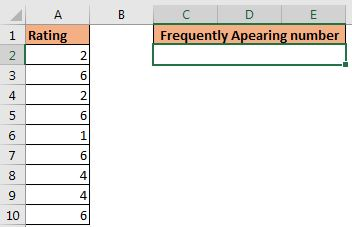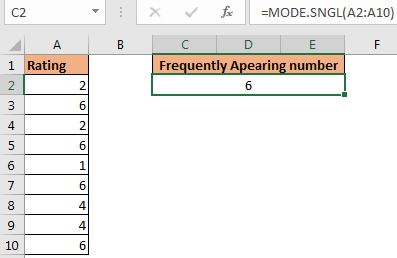# How to Use MODE.SNGL function

The MODE.SNGL function is a replacement of the MODE function in Excel. It was introduced in Excel 2010 with MODE.MULT function. It simply returns the most frequently appearing number in a range or array.

Syntax of MODE.SNGL function

 =MODE.SNGL(numbers)

range: This can be a range or an array of numbers.

Example: Get the most frequently appearing number using the MODE.MULT function.

Here I have a small list of numbers that represent ranks given by users of services. I want to look up the frequently given rank.Let's use the MODE.SNGL function to do so.

 =MODE.SNGL(A2:A10)How does it work?

This work the same as the MODE function. The question is why excel has introduced this new function. Actually, the MODE function only returns the single most frequently appearing number on the list. If there were two numbers with the same number of frequency, excel returns the first found the number. To solve this excel introduced MODE.MULT function to return multiple MODE values of the list and along with that, they introduced MODE.SNGL function works the same as MODE function but it is faster.

Notes:

1. The MODE.SNGL function returns the first number in the array with most frequency.
2. The function return #N/A error if there is no duplicate number in the range.
3. It ignores any text and blank cells in the range.

So yeah guys, this how you use MODE.SNGL function of excel. It was quite simple, but still, if you have any queries or special requirements in excel, ask in the comments section below. See you next time.

Related Articles:

How to Use Excel MODE.MULT function | Learn how to get multiple numbers with the most frequency in the range.

Excel MODE function | Use MODE function to get the most frequently appearing number in the range. It is a statistical function that helps in statistical analysis.

Get most frequently appearing text in Excel | Learn how to get frequently appearing text in excel.

Lookup Frequently Appearing Text with Criteria in Excel | To get the most frequently appearing text in excel with criteria you just need to put an if condition before match function.

Retrieving the First Value in a List that is Greater / Smaller than a Specified Value | Learn how to lookup first value that is greater than a specified value. The generic formula to lookup is...

How to retrieve the entire row of a matched value| It is easy to look up and retrieve the entire row from a table. Let's see how can we do this lookup.

How to Retrieve Latest Price in Excel | To look up the latest price from a range you must have your data sorted. Once sorted use this formula...

Popular Articles:

50 Excel Shortcuts to Increase Your Productivity | Get faster at your task. These 50 shortcuts will make your work even faster on Excel.

The VLOOKUP Function in Excel | This is one of the most used and popular functions of excel that is used to lookup value from different ranges and sheets.

COUNTIF in Excel 2016 | Count values with conditions using this amazing function. You don't need to filter your data to count specific value. Countif function is essential to prepare your dashboard.

How to Use the SUMIF Function in Excel | This is another dashboard essential function. This helps you sum up values on specific conditions.

Terms and Conditions of use

The applications/code on this site are distributed as is and without warranties or liability. In no event shall the owner of the copyrights, or the authors of the applications/code be liable for any loss of profit, any problems or any damage resulting from the use or evaluation of the applications/code.